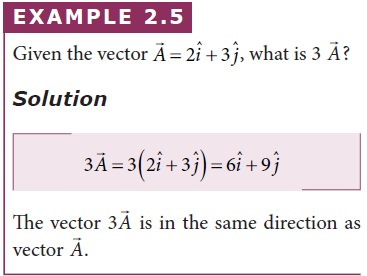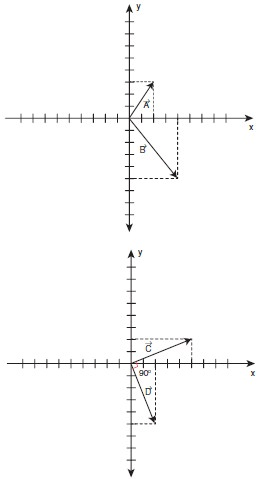Home | | Physics 11th std | Scalar Product of Two Vectors

# Scalar Product of Two Vectors

The scalar product (or dot product) of two vectors is defined as the product of the magnitudes of both the vectors and the cosine of the angle between them.

Scalar Product of Two Vectors

## Definition

The scalar product (or dot product) of two vectors is defined as the product of the magnitudes of both the vectors and the cosine of the angle between them.

Thus if there are two vectorsandhaving an angle θ between them, then their scalar product is defined as= AB cos θ. Here, A and B are magnitudes ofand.

## Properties

The product quantityis always a scalar. It is positive if the angle between the vectors is acute (i.e., < 90°) and negative if the angle between them is obtuse (i.e. 90°<θ< 180°).The work done is basically a scalar product between the force vector and the displacement vector. Apart from work done, there are other physical quantities which are also defined through scalar products.

## Solved Example Problems for Multiplication Of Vector By A Scalar## Solved Example Problems for Scalar Product of Two VectorsTags : Definition, Properties, Solved Example Problems , 11th Physics : UNIT 2 : Kinematics
Study Material, Lecturing Notes, Assignment, Reference, Wiki description explanation, brief detail
11th Physics : UNIT 2 : Kinematics : Scalar Product of Two Vectors | Definition, Properties, Solved Example Problems